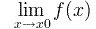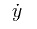# Calculus Symbols List: How to Read Equations

Calculus is a unique branch of mathematics, and includes many symbols and equations that are also unique. Some are intuitive and make sense at a glance, but others can be very confusing if they are new to you. Here is a quick overview of some of the calculus symbols you will come across.

## 1. Calculus Symbols: DerivativesThis is the format for writing a limit in calculus. When read aloud, it says “The limit of the function f of x, as x tends to 0.” (See: What is a limit?)

## f'(x)

This is a common symbol indicating the derivative of the function f(x). It reads simply as “The derivative of f of x.” (See: What is a derivative?)

## dy/dx

This is another symbol for a derivative. You can read it as “The derivative of y with respect to x.” Y is equivalent to f(x), as y is a function of x itself.

## f′′(x), d2y/dx

Both of these symbols represent the second derivative of the function, which means you take the derivative of the first derivative of the function. You would read it simply as “The second derivative of f of x.”

## fn(x), dn * y/dx

These symbols represent the nth derivative of f(x). Much like the second derivative, you would perform differentiation on the formula for n successive times. It reads as “The nth derivative of f of x.” If n were 4, it would be “The fourth derivative of x,” for example.This is the symbol for differentiation with respect to time. You can read it as “the derivative of y with respect to time.”
This is called dot notation; more dots indicate higher orders of differentiation. For example:2 dots = second derivative.

## 2. Calculus Symbols: IntegralsThis symbol represents integration of the function. Integration of a function is the opposite of the differentiation. The variables a and b represent the lower limit and upper limit of the section of the graph the integral is being applied to. If there are no values for a and b, it represents the entire function. You would read it as “The integral of f of x with respect to x (over the domain of a to b.)”

## Other Calculus Symbols

Symbol Name Meaning Example
x * y convolution y(t) = x(t)
* h(t)

ε epsilon a very small number, close to zero ε 0
e Euler’s number e = 2.718281828… e = lim (1+1/x)x
, x→∞
[a,b] closed interval [a,b] = {x | a x b}
(a,b) open interval (a,b) = {x | a < x < b}
i imaginary number i ≡ √-1 z = 4 + 2ivector
Infinity
! Factorial

## Delta

Delta is the fourth letter in the Greek alphabet.  It has different meanings depending on whether it appears in upper or lowercase form.

## Upper-Case (Δ)

Upper-case Δ has two different meanings.

1. A difference, or change, in a quantity:  For example , where we say “delta x” we mean how much x changes. You will often come across delta in this context when working with values that characteristically change, such as velocity or acceleration.  We also see this meaning when working with slope; The slope is the ratio of the vertical and horizontal changes between two points on a line. You’ll see the use of upper-case delta in the formula for slope: Slope = rise / run = Δy/Δx.
Other examples:

• Δt (“delta t“) = a small amount of time after t or before t,
• f(t + Δt) (“f of t plus delta t“) = the value of f at time t, plus a small amount of time after t or before t
• Δ f (“delta f“) = the change f(t + Δt) – f(t)
• Δf/Δt (“d f d t“) = average velocity
2. Discriminant:  This is much less common than the first meaning, but you might come across it if you’re working with the quadratic formula

## Lower-Case (δ) and the Epsilon-Delta Limit DefinitionWhenever a point x is within δ units of c, f(x) is within ε units of LLower-case δ is used when calculating limits.  The epsilon-delta definition of a limit is a precise method of evaluating the limit of a function.  Epsilon (ε) in calculus terms means a very small, positive number.  The epsilon-delta definition tells us that:

Where f(x) is a function defined on an interval around x0, the limit of f(x) as x approaches x0 is L.  For every ε > 0 there exists δ > 0 such that for all x:

0 <│x – x0│<  δ  →│f(x) – L│ <  ε

This definition is particularly useful; It makes sure that values returned by the function f(x) are as close to the limit as possible by only using points in a small interval around x0.  It gives us a useful measure regardless of how close to L we wish f(x) to be.

This is just a small calculus symbols list, but should provide a decent launching point for being able to understand calculus symbols and equations.

## References

Strang, G. (1991). Calculus: Volume 1. Siam.

CITE THIS AS:
Stephanie Glen. "Calculus Symbols List: How to Read Equations" From StatisticsHowTo.com: Elementary Statistics for the rest of us! https://www.statisticshowto.com/calculus-symbols-list-how-to-read-equations/